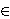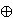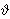### Sibe Mardešić

Department of Mathematics, University of Zagreb, Bijenička cesta 30, 10002 Zagreb, P.O. Box 335, Croatia
e-mail: smardes@math.hr

Abstract.   In a previous paper the author has associated with every inverse system of compact CW-complexes X with limit X and every simplicial complex K with geometric realization |K| a resolution of X × |K|, which consists of spaces having the homotopy type of polyhedra. In a subsequent paper it is shown that this construction is functorial. The proof depends essentially on particular cellular subdivisions of K. The purpose of this paper is to describe in detail these subdivisions and establish their relevant properties. In particular, one defines two subdivisions L(K) and N(K) of K. Each cell from L(K), respectively from N(K), is contained in a simplex σK and it is the direct sum ab, respectively cd, of certain simplices contained in σ. One defines new subdivisions L'(K) and N'(K) of K by taking for their cells the direct sums L(a)b, respectively cN(d). The main result asserts that there is an isomorphism of cellular complexes: L'(K) → N'(K), which induces a selfhomeomorphism θ : |K| → |K|.

2000 Mathematics Subject Classification.   55U10, 52B11, 54C56.

Key words and phrases.   Convex polytope, simplicial complex, cellular complex, subdivision of a complex, isomorphism of cellular complexes, resolution of a space, shape.

Full text (PDF) (free access)

DOI: 10.3336/gm.45.1.14

References:

1. P. Alexandroff and H. Hopf, Topologie, Springer, Berlin, 1935.

2. A. Brondsted, An introduction to convex polytopes, Graduate Texts in Mathematics, Springer, Berlin, 1983.
MathSciNet

3. J. Dugundji, Topology, Allyn and Bacon, Boston, 1966.
MathSciNet

4. B. Grünbaum, Convex polytopes, Interscience Publishers John Wiley & Sons, Inc., New York, 1967.
MathSciNet

5. S. Mardesic, Strong shape and homology, Springer Monographs in Mathematics, Springer-Verlag, Berlin, 2000.
MathSciNet

6. S. Mardesic, A resolution for the product of a compactum with a polyhedron, Topology Appl. 133 (2003), 37-63.
MathSciNet     CrossRef

7. S. Mardesic, Functoriality of the standard resolution of the Cartesian product of a compactum and a polyhedron, Topology Appl. 155 (2007), 1-32.
MathSciNet     CrossRef

8. S. Mardesic, J. Segal, Shape theory, North-Holland, Amsterdam, 1982.
MathSciNet

9. M. Moszynska, Selected topics in convex geometry, Birkhäuser, Basel, 2006.
MathSciNet